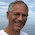## onsdag 25 november 2015

### New Proof of Planck's Radiation Law vs (Italian) Piano Tuning

Computation Blackbody Radiation presentats a new approach to Planck's radiation law based on finite precision computation applied to a wave model consisting a set of harmonic oscillators with small damping subject to near-resonant forcing, each one of the oscillators of the form
• $\ddot u(t)+\nu^2u(t)+\gamma\dot u(t)=f(t)$ for time $t>0$,
where $\dot u=\frac{du}{dt}$ and $\ddot u=\frac{d^2u}{dt^2}$, $\nu >> 1$ is the eigenfrequency of the oscillator, $\gamma$ is a small positive damping coefficient with $\gamma\nu\le 1$, and $f(t)$ is a near-resonant forcing, for example given by
• $f(t)=\sin((\nu -0.5)t)+\sin(\nu t)+\sin((\nu +0.5)t)$
with a total frequency shift of 1 Hz.

A basic aspect of this model connects to the so called Italian tuning of a piano, where the three strings in the middle high register for each key/tone are tuned with a total frequency shift of about 1 Hz as above.

Let us now seek to understand in what sense Italian tuning is different from standard tuning with the three strings for each key/tone tuned to exactly the same frequency or pitch. We then identify in the above model
• the three strings are represented by the forcing $f(t)$,
• the sound board of the piano is represented by the oscillator,
• the outgoing sound from the sound board is represented by the damping.
Let us first consider standard tuning with strings and soundboard all with the same eigen-frequency, that is a case with perfect resonance. A pressed key activates a hammer with hits the strings which start to vibrate and thereby as forcing transfers energy to the sound board, which in turn starts to vibrate and produces a sound. In this case the forcing $f(t)$ will stay in phase with the velocity $\dot u$ over time, which means quick transfer of energy from strings to sound board as the integral of the positive product $f(t)\dot u(t)$ as the work performed per unit time. The result may be an outgoing sound of relatively high volume but short duration.

On the other hand, with the Italian tuning, the forcing from each of the three strings with slightly different frequencies cannot all be in phase with the common sound board velocity $\dot u$ over time, which means less quick energy transfer to the sound board with $f(t)\dot u(t)$ of changing sign and thus slower string energy loss as compared to the standard case. The result may be a sound of less volume but longer duration (sustain) than in the standard case, and also with slight "beat".

Of course, in reality it may be difficult to clearly separate the two cases, because perfect resonance does not really occur for a real piano with standard tuning, because of the complexity of the sound board, and also because the damper stops the string vibration before the tone has faded.

In any case, the distinction between perfect and near resonance is fundamental in the new proof of Planck's radiation law offered as by Computation Blackbody Radiation, a proof without reference to mystical statistics.

PS It is possible to change the setting by letting $f(t)$ represent the outgoing sound and the damping the input from the strings to the sound board. This is of relevance in stationary periodic state with sustained sound over long time without damping.

#### 1 kommentar:

1.This is a really nice and understandable illustration on a topic that has been mystified and covered up in photon fog by "climate science"